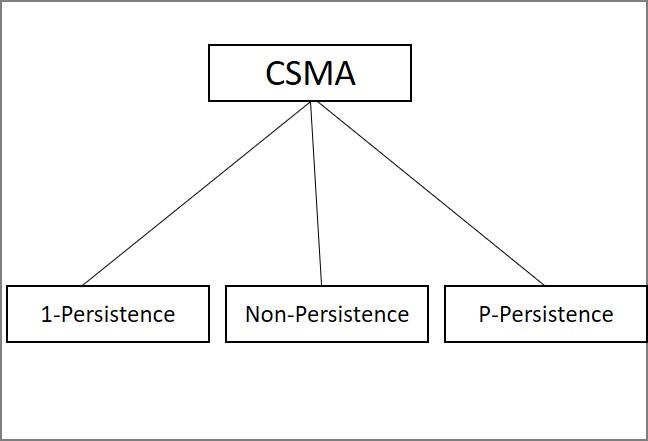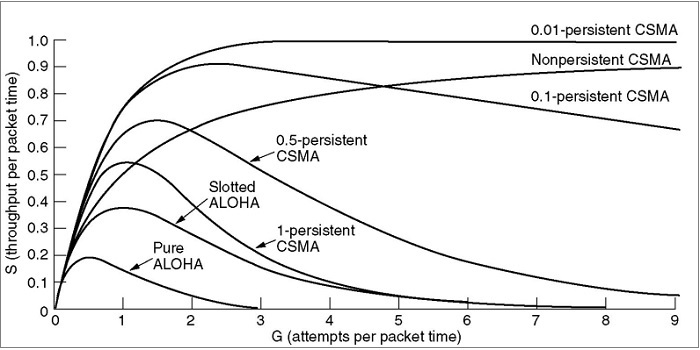# What is carrier sense multiple access protocols?

Protocols in which stations listen for a carrier and act accordingly are called Carrier Sense Protocols. Multiple Access tells the fact that multiple nodes send and receive on the medium.

Transmissions of one node are received by all other nodes using the medium. Carrier Sense Multiple Access (CSMA) is a probabilistic Media Access Control (MAC) protocol in which a node verifies the absence of other traffic before transmitting on a shared physical medium, such as an electrical bus, or a band of electromagnetic spectrum.

Carrier Sense Multiple Access (CSMA) Protocols

The three protocols that discuss the various implementations are as follows −

• 1-persistent CSMA
• Non- Persistent CSMA
• p-persistent CSMA## 1-persistent CSMA

The 1-Persistent CSMA is explained below in a stepwise manner.

Step 1 − When a node has data to send, it first listens to the channel to see if anyone is transmitting.

Step 2 − If the channel is busy, the station waits until it becomes idle.

Step 3 − When the station identifies an idle channel, it transmits a frame.

Step 4 − If a collision occurs, the station waits a random amount of time and starts retransmission.

The only drawback is that the propagation delay time affects the 1-persistent CSMA protocol.

## Example

Let us consider an example, just after station A begins its transmission, station B also becomes ready to send its data and senses the channel. If the station A signal has not yet reached station B, station B will sense the channel to be idle and will begin its transmission. This will result in a collision.

Even if propagation delay time is zero, then also collision occurs. If two stations become ready in the middle of the third station’s transmission, both stations will wait until the transmission of the first station ends and then both will begin their transmission exactly simultaneously. This will also result in collision.

## Non-persistence CSMA

The Non-Persistence CSMA is explained below in a stepwise manner.

Step 1 − An attempt is made to be less greedy than persistence.

Step 2 − A node that has a frame to send first senses the channel. If the channel is idle, then it senses immediately.

Step 3 − If the channel is busy, then it waits for a random amount of time and then senses the channel again.

Step 4 − In non-persistence CSMA the station does not continuously sense the channel for the purpose of capturing it when it detects the end of previous transmission.

Step 5 − Consequently this Non persistence leads to better channel utilisation but longer delays than 1-persistence CSMA. Here, the number of collisions is reduced.

The advantage is that it reduces the chances of collision because the nodes wait for a random amount of time before transmitting.

The disadvantage is that it reduces the efficiency of the network because the channel remains idle when there may be stations with frames to send. This is because the nodes are waiting a random amount of time before transmitting.

## P-Persistence

The P-Persistence CSMA is explained below in a stepwise manner.

Step 1 − It applies to slotted channels so that the time slot duration is equal to or greater than maximum propagation delay time.

Step 2 − When a station becomes ready to send, it senses the channel.

Step 3 − If it is idle, it transmits with a probability p. with probability q=1-p, it defers until the next slot.

Step 4 − If that slot is idle it either transmits or defers again, with probability p and q. This process is repeated until either the frame has been transmitted or another node has begun transmitting.

Step 5 − In the latter case, the node acts as if there had been a collision

Step 6 − If the station initially senses that the channel is busy, it waits until the next slot and applies the above algorithm.

Step 7 − IEEE 802.11 uses refinement of p-persistence CSMA.

The advantages are that the P-Persistence reduces the chances of collision and improves the efficiency of the network.

Let’s see the comparison of the channel utilization versus load for various random-access protocols −